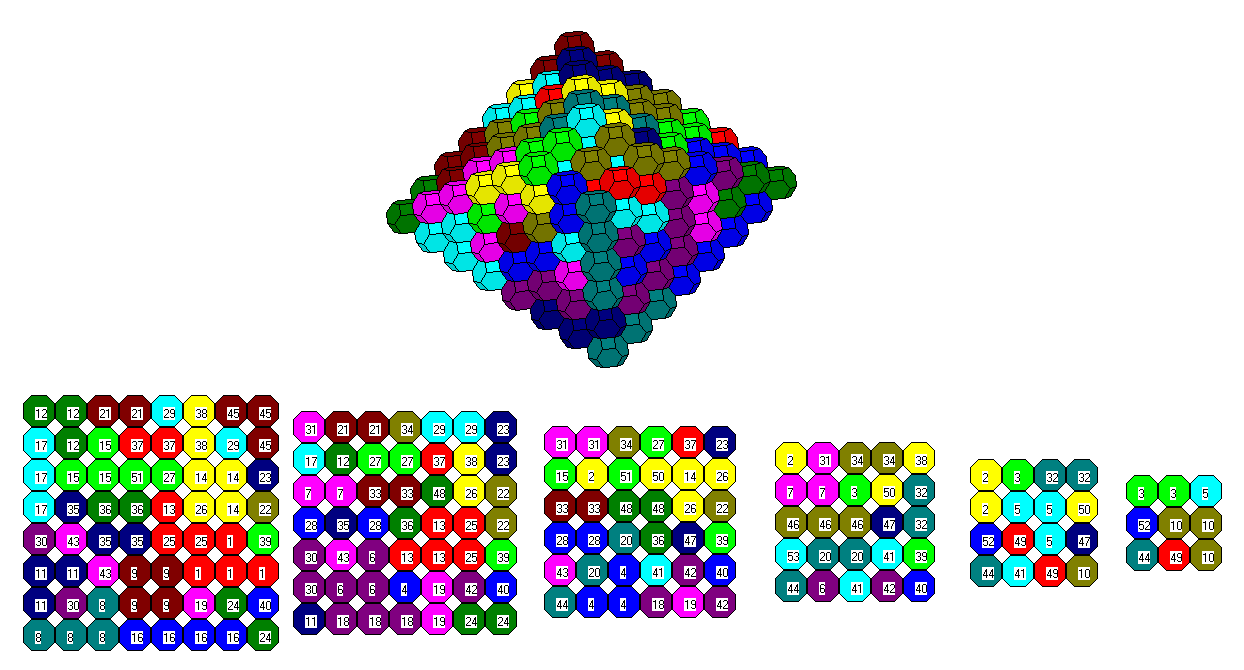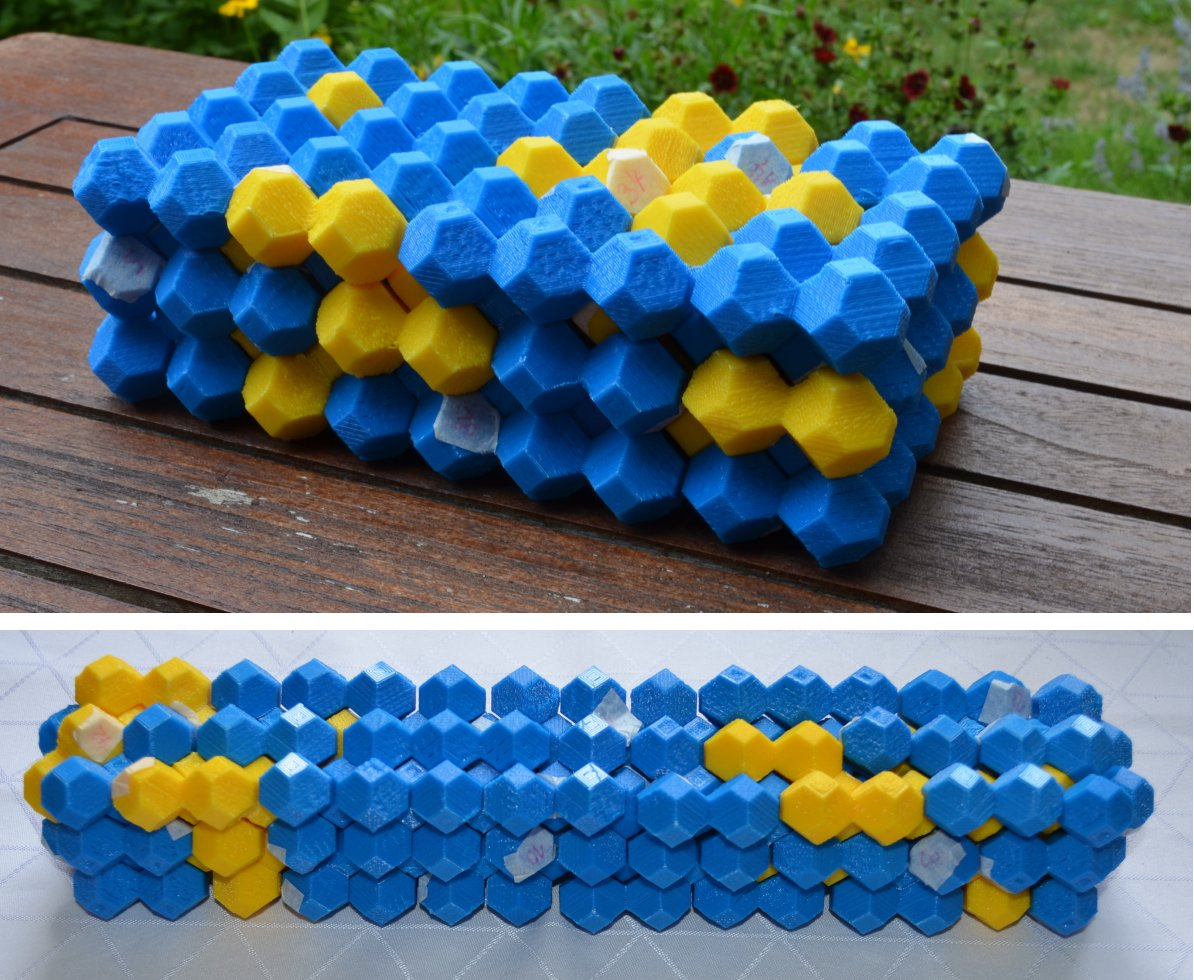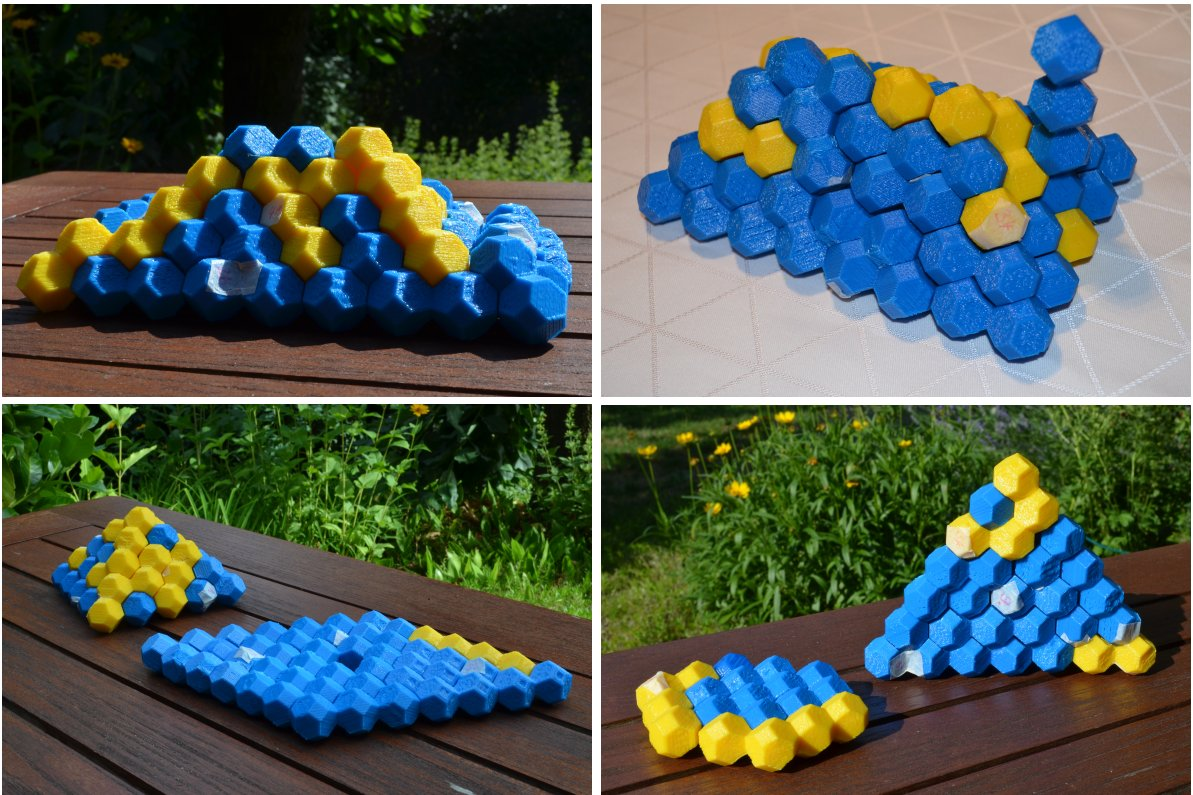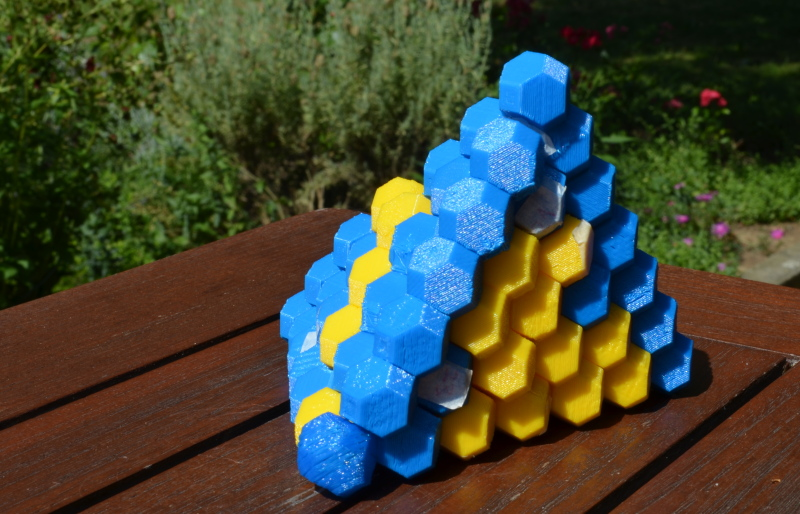# Polytrocs of Order 1..4

Taking all polytrocs of order 4 or less we get 53 pieces with a total volume of 199 and the nasty problem with an odd number of odd tetratrocs is gone.

Here are the small polytrocs numbered from 45 to 53.The truncated square pyramid is already shown in the title.Two boxes with five layers each can also be constructed.Since all 9 polytrocs of order 3 or less are planar we have 20+9=29 planar pieces with a total volume of 103. This allows for a roof with a step or a chimney and two combined constructions.Among the 29 planar pieces there are 20+4=24 which fit the hexagonal grid. This is just enough for a tetrahedron of size 7.You can also solve some flat figures.If you left over the three pieces of order 1 and 2 you can get some hexagonsBack
Home